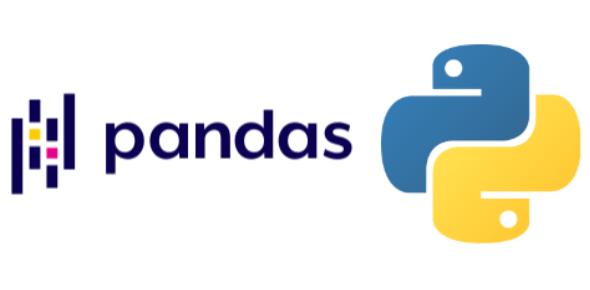# Programming Quiz: Pandas Software Library

10 Questions | Total Attempts: 191SettingsIf you are into computer programming, we assume you are familiar with Pandas software library. Here are some questions related to its operational use, syntax, structure, etc. So, without further ado, let's get started.

• 1.
CSV refers to tabular data saved as plain text where data values are separated by commas.
• A.

True

• B.

False

• 2.
Read_csv() method automatically takes the last row of the CSV file.
• A.

True

• B.

False

• 3.
Full form of csv is common separated value.
• A.

True

• B.

False

• 4.
DataFrame in pandas is:
• A.

1 dimensional array

• B.

2 dimensional array

• C.

3 dimensional array

• D.

None of these

• 5.
Series in pandas is:
• A.

1 dimensional array

• B.

2 dimensional array

• C.

3 dimensional array

• D.

None of these

• 6.
We can analyze the data in pandas with:
• A.

Series

• B.

DataFrame

• C.

Both of these

• D.

None of these

• 7.
import pandas as pd s = pd.Series([1,2,3,4,5],index = ['a','b','c','d','e']) print s['a']
• A.

1

• B.

2

• C.

3

• D.

4

• 8.
Which of the following thing can be data in Pandas?
• A.

A python dict

• B.

an ndarray

• C.

A scalar value

• D.

All of these

• 9.
Pandas is an open-source _______ Library?
• A.

Ruby

• B.

Javascript

• C.

Java

• D.

Python

• 10.
Which of the following is correct Features of DataFrame?
• A.

Potentially columns are of different types

• B.

Can Perform Arithmetic operations on rows and columns

• C.

Labeled axes (rows and columns)

• D.

All of the above

Related TopicsBack to top